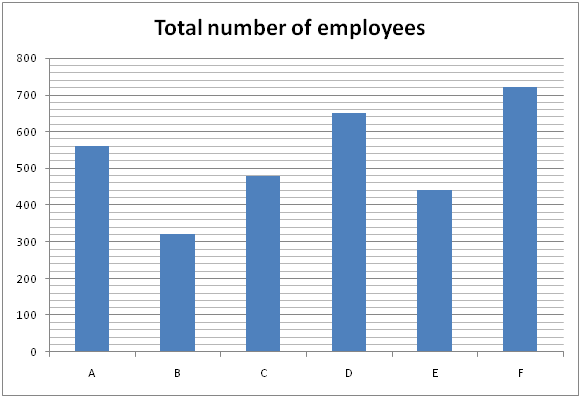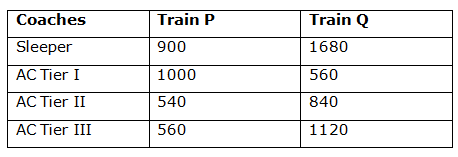# “The Knowledge Library”

Knowledge for All, without Barriers…

# “The Knowledge Library”

Knowledge for All, without Barriers……….

# Data Interpretation-12

Directions (Q. 1 – 5): Study the following information carefully and answer the given questions.

The following bar graph shows the total number of employees (Officers and clerks) in 6 different companies in a certain year.1) If the ratio between the total number of officers to that of clerks in the company A and C is 3 : 5 and 3 : 13 respectively and the percentage of clerks in the company B is 80 %, then find the sum of the total number of officers in the company A, B and C together?

a) 382

b) 350

c) 336

d) 364

e) None of these

2) If the ratio between the total number of officers to that of clerks in the company D is 4 : 9 and the total number of male officers in company D is 60 % of total number of female officers, then the total number of clerks in company D is what percentage of total number of female officers in company D?

a) 280 %

b) 360 %

c) 325 %

d) 375 %

e) None of these

3) Find the average number of employees in all the given companies together except company D?

a) 504

b) 536

c) 488

d) 472

e) None of these

4) If the ratio between the total number of officers to that of clerks in company E is 4 : 7 and the percentage of total number of officers in company F is 30 %, then find the difference between the total number of clerks in company E to that of company F?

a) 286

b) 318

c) 224

d) 352

e) None of these

5) Total number of employees in company A, C and E together is approximately what percentage of total number of employees in company B, D and F together?

a) 95 %

b) 88 %

c) 112 %

d) 73 %

e) 66 %

Caselet

Directions (Q. 6 – 10): Study the following information carefully and answer the given questions?

There are two trains, Train P and Train Q. Both the trains have four different types of coaches, viz Sleeper, AC Tier III, Tier II and Tier I. In train P, there are total of 3000 passengers. Train Q has 40 % more passengers than Train P. 30 % of the passengers in Train P are in sleeper coaches. One – third of the total number of passengers in Train P are in AC Tier I. 18 % of the passengers in Train P are in AC Tier II and the remaining are in AC Tier III. 40 % of the total number of passengers of Train Q are in Sleeper coaches. In Train Q, the ratio of the passengers of AC Tier III, Tier II and Tier I is 4: 3: 2.

6) The total number of passengers in Sleeper class in both the trains together is what percentage of total number of passengers in Train Q?

a) 44 %

b) 28 %

c) 75 %

d) 61 %

e) 83 %

7) The costs per ticket of train P for AC tier III and Tier I are Rs. 1800 and Rs. 3000 respectively. Then find the total amount generated by both the coaches in Train P?

a) Rs. 4008000

b) Rs. 5156000

c) Rs. 4524000

d) Rs. 4872000

e) None of these

8) Find the difference between the average number of passengers in AC Tier I, II and III together in train Q to that of total number of passengers in AC tier II and III together in train P?

a) 380

b) 450

c) 260

d) 320

e) None of these

9) Find the ratio between the total number of passengers in Sleeper and AC tier I together in train P to that of total number of passengers in AC tier II and tier III together in train Q?

a) 52: 59

b) 95: 98

c) 43: 51

d) 29: 33

e) None of these

10) Total number of passengers in AC tier II in both the trains together is approximately what percentage more/less than the total number of passengers in AC tier III in both the trains together?

a) 18 % less

b) 32 % more

c) 18 % more

d) 32 % less

e) 45 % more

Directions (1-5) :

The ratio between the total number of officers to that of clerks in the company A = 3 : 5

The ratio between the total number of officers to that of clerks in the company C = 3 : 13

The percentage of clerks in the company B = 80 %

The total number of officers in the company A, B and C together

= > (560/8) * 3 + (480/16) * 3 + 320 * (20/100)

= > 210 + 90 + 64 = 364

The ratio between the total number of officers to that of clerks in the company D = 4 : 9

The total number of male officers in company D = 60 % of total number of female officers

Male officers = (60/100)*Female officers

Male officers : Female officers = 3 : 5

The total number of clerks in company D = (650/13)*9 = 450

The total number of officers in company D = 650 – 450 = 200

The total number of female officers in company D

= > (200/8)*5 = 125

Required % = (450/125)*100 = 360 %

The average number of employees in all the given companies together except company D

= > (560 + 320 + 480 + 440 + 720)/5

= > 2520/5 = 504

The ratio between the total number of officers to that of clerks in company E

= 4 : 7

The percentage of total number of officers in company F = 70 %

The difference between the total number of clerks in company E to that of company F

= > 720*(70/100) – (440/11)*7

= > 504 – 280 = 224

Total number of employees in company A, C and E together

= > (560 + 480 + 440) = 1480

Total number of employees in company B, D and F together

= > (320 + 650 + 720) = 1690

Required % = (1480/1690)*100 = 87.57 % = 88 %

Directions (Q. 6 – 10):

Total passengers in train P = 3000

Total passengers in train Q = 3000 * (140/100) = 4200

Total passengers in Sleeper coaches in train P = 3000 * (30/100) = 900

Total passengers in AC Tier I in train P = 3000 * (1/3) = 1000

Total passengers in AC Tier II in train P = 3000 * (18/100) = 540

Total passengers in AC Tier III in train P

= > 3000 – (900 + 1000 + 540) = 560

Total passengers in Sleeper coaches in train Q = 4200 * (40/100) = 1680

Total passengers in AC Tier I in train Q = 2520 * (2/9) = 560

Total passengers in AC Tier II in train Q = 2520 * (3/9) = 840

Total passengers in AC Tier III in train Q = 2520 * (4/9) = 1120The total number of passengers in Sleeper class in both the trains together

= > 900 + 1680 = 2580

The total number of passengers in Train Q = 4200

Required % = (2580 / 4200) * 100 = 61.428 % = 61 %

The total number of passengers in AC Tier III in train P = 560

The total number of passengers in AC Tier I in train P = 1000

The total amount generated by both the coaches in Train P

= > 560 * 1800 + 1000 * 3000

= > 1008000 + 3000000 = Rs. 4008000

The average number of passengers in AC Tier I, II and III together in train Q

= > (560 + 840 + 1120)/3

= > 2520/3 = 840

The total number of passengers in AC tier II and III together in train P

= > 540 + 560 = 1100

Required difference = 1100 – 840 = 260

The total number of passengers in Sleeper and AC tier I together in train P

= > 900 + 1000 = 1900

The total number of passengers in AC tier II and tier III together in train Q

= > 840 + 1120 = 1960

Required ratio = 1900: 1960 = 95: 98

Total number of passengers in AC tier II in both the trains together

= > 540 + 840 = 1380

Total number of passengers in AC tier III in both the trains together

= > 560 + 1120 = 1680

Required % = [(1680 – 1380) / 1680] * 100 = 17.85 % = 18 % less